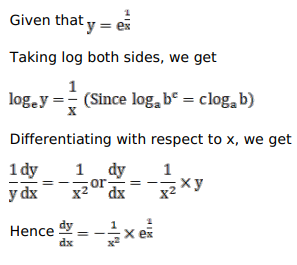# Solve this followingQuestion:

Mark $(\sqrt{ })$ against the correct answer in the following:

If $y=e^{1 / x}$ then $\frac{d y}{d x}=$ ?

A. $\frac{1}{\mathrm{x}} \cdot \mathrm{e}^{(1 / \mathrm{x}-1)}$

B. $\frac{-e^{1 / x}}{x^{2}}$

C. $e^{1 / x} \log x$

D. none of these

Solution: